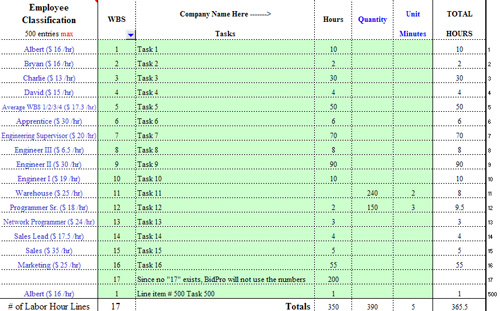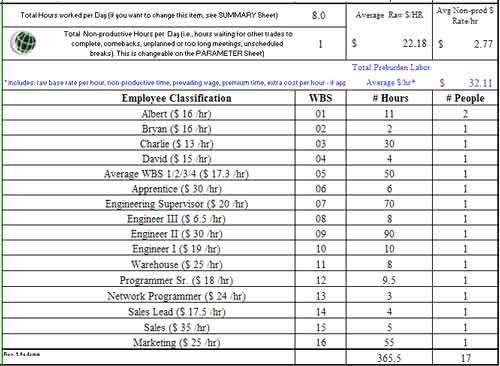BidQwik.com

Labor Sheet

The Labor Sheet has 500 lines to accommodate labor activities or tasks. The WBS entry from the Parameter Sheet will depict the user set Classification for the worker and the corresponding pay rate. Note, only the dollar values under “This Bid Raw \$” will be utilized in labor rate calculations.

By placing a 1 (or 01) on line 1 of the WBS column Labor Worksheet, BidPro will place Albert on the corresponding Employee Classification line. You may now describe the task and associated time it takes to do this task. BidPro knows the pay scale of that person (or a classification like Engineer).

Figure 1 Labor Tasks (up to 500 line items)By defining the tasks to take 2 minutes each, BidPro calculates the 240 "Task 11" will take 8.0 hours and averages all the Raw Labor Rates at the sheet bottom. In this example, the average labor rate would be \$21.36 per hour for the assigned 16 workers (note Albert has 2 Tasks). Note, the Total Hours worked portion indicates 8.0, this comes from the Summary Sheet and signifies the workday is 8 hours per day…more on that later.

The Avg Non-prod \$ Rate/hr (Average Non-productive Labor Rate per hour) is calculated for each WBS line item placed on the Labor Sheet. If Albert works a full day (8 hrs x \$16/hr), his pay would be \$128. However, if we planned a task to take 8 hours, Albert would do this in 9 hours since he takes a 15-minute break every 2 hours for whatever reason (15 minutes x 4 breaks/day = 1 hour). Without considering overtime, BidPro calculates this task will cost \$144 (9 hours x \$16/hr). The difference between \$144.00 and \$128.00 hours is \$16.00. This difference of \$16 over 8 hours averages to \$2.00 per hour. The non-productive time will be listed as \$2.00 on the Summary Sheet for Albert. Since this calculation adds a cost to our bottom line, it will also reflect an increase in sell price. BidPro has calculated The Average Raw Labor Rate/Hour is \$22.18 and the Non-productive \$Rate/Hour at \$2.77. This amount is summarized as the Total Preburden Labor Average Rate/hour as \$32.11.

Note: the following table at the bottom of the Labor Sheet, Figure 41, will total all WBS lines and provides total hours and the number of times that WBS appears on the Labor Sheet.

Figure 2 WBS Labor Sheet BreakdownAs far as BidPro is concerned, there are 16 WBS categories and 17 workers (note Albert WBS shows 2 people because 2 lines for ALbert were used) that will do tasks for 365.5 hours. The 17 people will be used to average how a long a project will take to complete (Summary Sheet). It is on the Summary Sheet that additional refinements can be made to the project duration